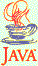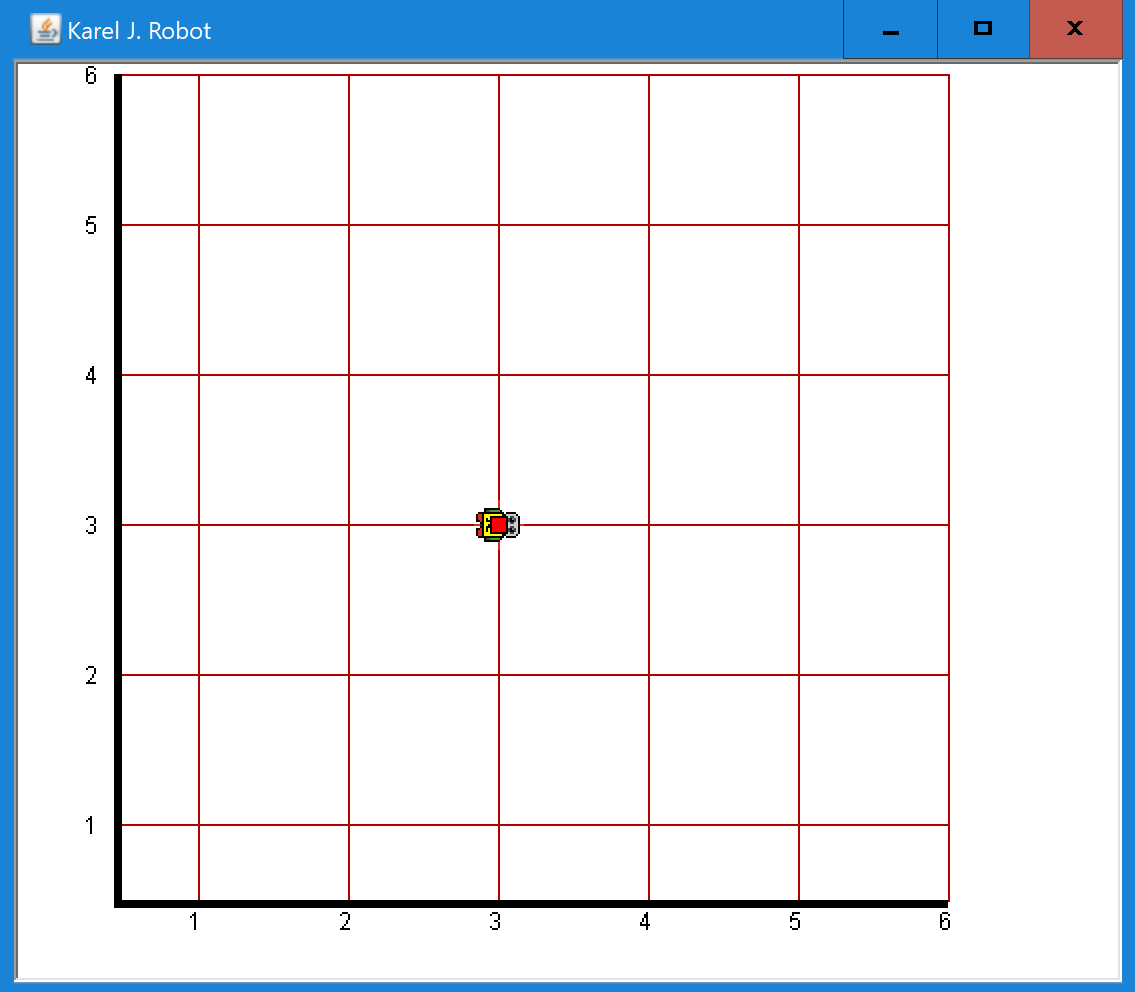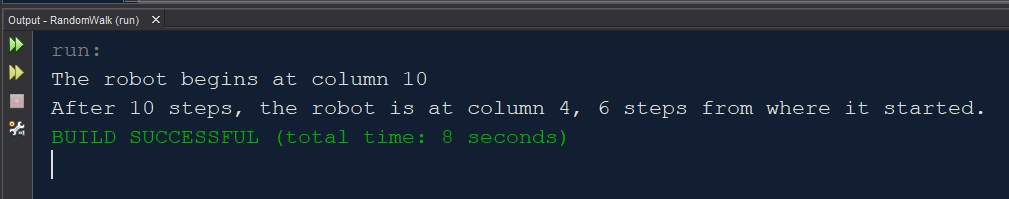### Random Walk5 points

In a random walk, a person (or here, a robot) starts at a given position. He then randomly takes 1 step to the left or 1 step to the right; the two possibilities are equally likely. In this random walk program, which uses Karel J. Robot, we look at how far the robot winds up from where he started.

What to you think will happen? Will the robot usually wind up pretty close to where he started? Will he wind up farther away from where he started if he moves a larger number of steps? To answer these questions, we'll run simulations.

Download RandomWalk.java below and complete the 'TO DO' sections. If you run the program with a starting position of (10, 10) and have the robot move 10 steps, you might get output like this screenshot:### Extra Credit: Many Simulations

3 points

Next we want to add the ability to run a simulation like this many times. In the ManageWalk constructor, set PRINTING to false to stop the program from executing 'System.out.println' commands. In main(), send 'false' to 'setTrace' to suppress Robot messages. Set the number of steps that the robot takes to 10. Add a loop to main() so that the simulation runs 1000 times. You will need to start the robot much farther to the right to avoid the robot dying when he tries to walk through the wall at the left side of the screen. I started the robot at column 250. In main(), use FileWriter to create an output file, and write to it, inside the loop, how far away the robot winds up from where it started (its displacement). I added a print statement inside the loop so that I could watch the program's progress:You will then have an output file with 1000 numbers. Open the file in Excel and find the average of the 1000 numbers. Then, in a second tab in Excel, start constructing a table that keeps track of how many steps the robot took in each simulation, and the robot's average displacement. In the first row of the table, the first entry will be 10 and the second entry will be the average you calculated above.

Run another set of 1000 simulations in which the number of steps the robot takes is 30. Again find the robot's average displacement and enter a second row in the table on the second Excel tab.

Do 1000 simulations with 100 robot steps, 300, 600 and 1000. Enter the resulting data in the table on the second tab.

You'll then have a table with 6 rows. Select the 6 rows. Then create a chart from the table: Insert - Scatter (X-Y). The chart can suggest how this function would grow if you continued the simulations with larger numbers of robot steps.